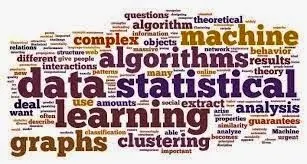# How to Check Multicollinearity in Categorical Variables

In regression models, it is required to meet the assumption of multicollinearity (or collinearity). Multicollinearity means Independent variables are highly correlated to each other.When multicollinearity is present, it can have several effects and implications on the regression analysis:

• `Unreliable Coefficient Estimates`: Multicollinearity makes it difficult for the model to determine the unique contribution of each independent variable. As a result, the coefficient estimates may become unstable and unreliable.
• `Difficulty in Interpretation`: With high multicollinearity, it becomes challenging to interpret the individual effects of independent variables on the dependent variable. It becomes unclear which variable is truly influencing the outcome, and the magnitude and direction of the effects may be distorted.
• `Inflated Standard Errors`: Multicollinearity inflates the standard errors of the regression coefficients. Larger standard errors indicate increased uncertainty in the estimates, making it harder to determine whether the coefficients are statistically significant or not.
• `Inconsistent Significance Tests`: Multicollinearity can lead to inconsistent results in significance tests for individual coefficients. Variables that might have been significant in a simple regression model could become non-significant in the presence of multicollinearity.

## Checking Multicollinearity in Categorical Variables

Please note that multicollinearity is measured by checking the relationship between two or more independent variables in the model. The dependent variable is not considered while measuring multicollinearity.

• When dealing with ordinal variables, multicollinearity can be detected with Spearman rank correlation coefficient. The Spearman rank correlation measures the strength and direction of the monotonic relationship between two ordinal variables. A high Spearman rank correlation coefficient between two ordinal predictors suggests a potential multicollinearity issue.
• When dealing with nominal variables, multicollinearity can be detected with chi-square test. The chi-square test measures the association between two categorical variables. If the chi-square test indicates a significant association between two nominal predictors, it may suggest the presence of multicollinearity.
• For a categorical and a continuous variable, multicollinearity can be measured using a t-test (if the categorical variable has 2 categories) or ANOVA (if it has more than 2 categories).
• Instead of using a categorical variable with "k" levels, you can create (k-1) dummy variables and then run the Variance Inflation Factor (VIF) on them to check multicollinearity. For example, you have a categorical variable called "marital status" which has 3 categories - Single, Married, Divorced. You can create 2 dummy variables which have either 0 or 1. Refer the table below. For a dummy variable "Single", it will have 1 against "single" of the original categorical variable, else 0 against the remaining categories. Similarly, for a dummy variable "Married", it will have 1 against "married" level, otherwise 0. In this case, we have set "Divorced" as reference level which means it will be 0 in both the dummy variables.
Learn how to create dummy variables
Marital Status Single Married
1 (Single) 1 0
2 (Married) 0 1
3 (Divorced) 0 0
Related Posts
ShareDeepanshu founded ListenData with a simple objective - Make analytics easy to understand and follow. He has over 10 years of experience in data science. During his tenure, he worked with global clients in various domains like Banking, Insurance, Private Equity, Telecom and HR.

6 Responses to "How to Check Multicollinearity in Categorical Variables"
1.Hi,

Thanks for your great work. Could please explain this in more detail. Like age group and income group. Both are co-related but which test we would we use in SAS to quantify this relationship.

2.One question
What test is applicable if checking multicollinearity between cateogrical and continuous variable.

3.If both dependent and independent variable are categorical ,how can multicollinarity test be done?

1.Multicollinearity means "Independent variables are highly correlated to each other".
your response or dependant variable is not considered while checking multicollinearity.

4.If two categorical variables are significantly associated, can we use both variables in a logistic regression model?

1.If two categorical variables are significantly associated, you should not use both in logistic regression model. This is because one of the assumption of the logistic regression model is 'absence of multi-collinearity' among it's independent features. Hence use either of it or feature engineer new variable by using these both.

Next → ← Prev